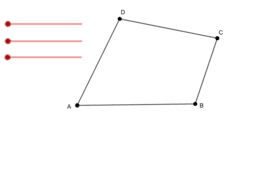# Sum of Interior Angles in a Polygon

Author:
caydenja
Topic:
Angles
A student-based discovery activity that explores the sum of the interior angles of a polygon by deconstructing the polygons into triangles, and then calculating the sum of degrees for every triangle that could be made.